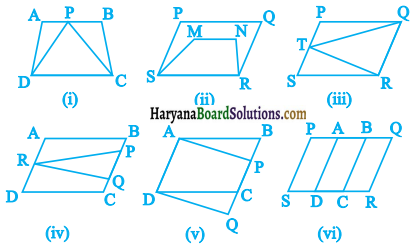# HBSE 9th Class Maths Solutions Chapter 9 Areas of Parallelograms and Triangles Ex 9.1

Haryana State Board HBSE 9th Class Maths Solutions Chapter 9 Areas of Parallelograms and Triangles Ex 9.1 Textbook Exercise Questions and Answers.

## Haryana Board 9th Class Maths Solutions Chapter 9 Areas of Parallelograms and Triangles Exercise 9.1

Question 1.
Which of the following figures lie on the same base and between the same parallels. In such a case, write the common base and the two parallels.Solution:
(i) The trapezium ABCD and ΔPDC are on the same base DC and lie between the same parallels AB and CD.
(ii) Parallelogram PQRS and trapezium MNRS are on the same base RS but they do not lie between the same parallels.
(iii) Triangle TQR and parallelogram PQRS are on the same base QR and lie between the same parallels PS and QR.
(iv) Triangle RPQ and parallelograms ABCD lie between the same parallels AD and BC but they are not on the same base.
(v) The parallelograms ABCD and APQD are on the same base AD and lie between the same parallels AD and BQ.
(vi) Parallelograms PQRS, AQRD, BQRC are not between the same parallels.